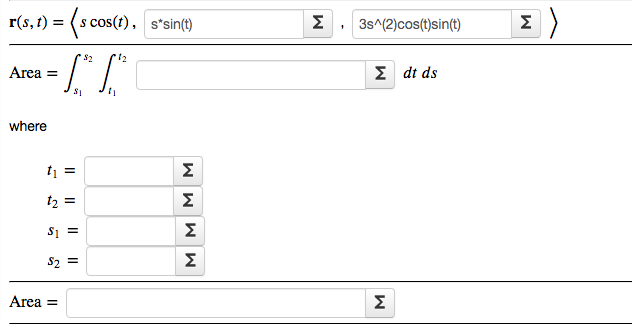# r(s, t) = (s cos(t), s*sin(t) Σ ) Σ 3s^(2)cos(t)sin(t) 12 Area = Σ dt ds where t1 = 12 = Σ Σ S2 = Σ Area = Σ M M M M

Question

Find the area of the part of the surface z=3xy that lies within the cylinder x^2+y^2 ≤ 4help_outlineImage Transcriptioncloser(s, t) = (s cos(t), s*sin(t) Σ ) Σ 3s^(2)cos(t)sin(t) 12 Area = Σ dt ds where t1 = 12 = Σ Σ S2 = Σ Area = Σ M M M M fullscreen

### Want to see this answer and more?

Experts are waiting 24/7 to provide step-by-step solutions in as fast as 30 minutes!*

*Response times may vary by subject and question complexity. Median response time is 34 minutes for paid subscribers and may be longer for promotional offers.
Tagged in
Math
Calculus

### Integration